# CLASS 8 MATHS CHAPTER 4 – PRACTICAL GEOMETRY

### Exercise 4.1

Question 1.
AB = 4.5 cm, BC = 5.5 cm, CD = 4 cm,
AD = 6 cm, AC = 7 cm
JU = 3.5 cm, UM = 4 cm, MP = 5 cm,
PJ = 4.5 cm, PU = 6.5 cm
(iii) Parallelogram MORE
OR = 6 cm, RE = 4.5 cm, EO = 7.5 cm
(iv) Rhombus BEST
BE = 4.5 cm, ET = 6 cm

Solution :

### NCERT Solutions for Class 8 Maths Exercise 4.2

Question 1.

LI = 4 cm, IF = 3 cm, TL = 2.5 cm, LF = 4.5 cm, IT = 4 cm
OL = 7.5 cm, GL = 6 cm, GD = 6 cm, LD = 5 cm, OD = 10 cm
(iii) Rhombus BEND
BN = 5.6 cm, DE = 6.5 cm

Solution :
(i) Given:; LI = 4 cm, IF = 3 cm, TL = 2.5 cm, LF = 4.5 cm, IT = 4 cm
Steps of construction: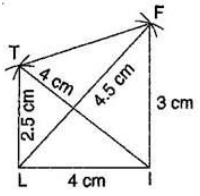(a) Draw a line segment LI = 4 cm.
(b) Taking radius 4.5 cm, draw an arc taking L as centre.
(c) Draw an arc of 3 cm taking I as centre which intersects the first arc at F.
(d) Join FI and FL.
(e) Draw another arc of radius 2.5 cm taking L as centre and 4 cm taking I as centre which intersect at T.
(f) Join TF, Tl and TI.
It is the required quadrilateral LIFT.
(ii) Given:; OL = 7.5 cm, GL = 6 cm, GD = 6 cm, LD = 5 cm, OD = 10 cm
Steps of construction: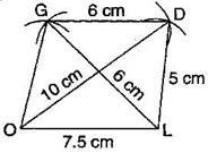(a) Draw a line segment OL = 7.5 cm
(b) Draw an arc of radius 5 cm taking L as centre and another arc of radius 10 cm taking O as centre which intersect the first arc point at D.
(c) Join LD and OD.
(d) Draw an arc of radius 6 cm from D and draw another arc of radius 6 cm taking L as centre, which intersects at G.
(e) Join GD and GO.
It is the required quadrilateral GOLD.
(iii) Given:; BN = 5.6 cm, DE = 6.5 cm
To construct: A rhombus BEND.
Steps of construction: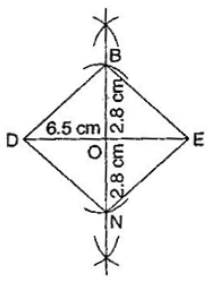(a) Draw DE = 6.5 cm.
(b) Draw perpendicular bisector of line segment DE.
(c) Draw two arcs of radius 2.8 cm from intersection point O, which intersects the line KN at B and N.
(d) Join BE, BD as well as ND and NE.
It is the required rhombus BEND.

NCERT Solutions for Class 8 Maths Exercise 4.3

MO = 6 cm, OR = 4.5 cm,
∠ M = 60; ∠ O =1050; ∠R =1050

PL = 4 cm, LA = 6.5 cm,
∠ P =900; ∠A = 1100; ∠N =850

(iii) Parallelogram HEAR
HE = 5 cm, EA = 6 cm, ∠ R =850

(iv) Rectangle OKAY
OK = 7 cm, KA = 5 cm

Solution :

(i) Given:; MO = 6 cm, OR = 4.5 cm,
∠ M = 600 ∠O =1050; ∠R =1050
Steps of construction:

(a) Draw a line segment MO = 6 cm.
(b) Construct ∠ R =; 105and taking radius 4.5 cm, draw an arc taking O as centre, which intersects at R.
(c) Also construct an angle; at R and 1050; produce the side RE.
(d) Construct another angle of; at point M and produce the side ME. Both sides ME and RE intersect at E.
It is the required quadrilateral MORE.
(ii) Given:; PL = 4 cm, LA = 6.5 cm,
∠ P = 900; ∠A = 110∠N =850
To find:; L =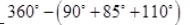Steps of construction: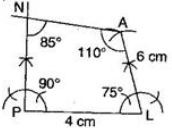(a) Draw a line segment PL = 4 cm.
(b) Construct angle of; at P and produce the side PN.
(c) Construct angle of; at L and with L as centre, draw an arc of radius 6 cm, which intersects at A.
(d) Construct A =; at A and produce the side AN which intersects PN at N.
It is the required quadrilateral PLAN.
;

(iii) Given:; HE = 5 cm, EA = 6 cm,
∠R =850
To construct: A parallelogram HEAR.
To find:; ∠H = 180º -85º = 95º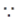Sum of adjacent angle of || gm is 180º ]
Steps of construction: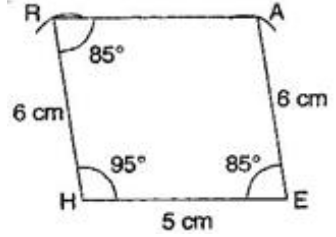(a) Draw a line segment HE = 5 cm.
(b) Construct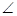H =; 95º and draw an arc of radius 6 cm with centre H. It intersects AR at R.
(c) Join RH.
(d) Draw ∠R = E = 85º and draw an arc of radius 6 cm with E as a centre which intersects RA at A.
(e) Join RA
It is the required parallelogram HEAR.
(iv) Given:; OK = 7 cm, KA = 5 cm
To construct: A rectangle OKAY.
Steps of construction:

(b) Construct angle; at both points O and K and produce these sides.
(c) Draw two arcs of radius 5 cm from points O and K respectively. These arcs intersect at Y and A.
(d) Join YA.
It is the required rectangle OKAY.

NCERT Solutions for Class 8 Maths Exercise 4.4

Draw the following:
Question 1.

The square READ with RE = 5.1 cm.

Solution :

Given:; RE = 5.1 cm.
Steps of construction: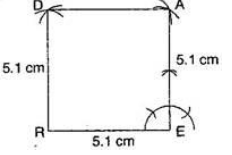(i) Draw RE = 5.1 cm.
(ii) At point E, construct an angle of; 90º and draw an arc of radius 5.1 cm, which intersects at point A.
(iii) At point R, draw an arc of radius 5.1 cm at point A, draw another arc of radius 5.1 cm which intersects the first arc at point D.
It is the required square READ.

Question 2.

A rhombus whose diagonals are 5.2 cm and 6.4 cm.

Solution :

Given:; Diagonals of a rhombus
AC = 5.2 cm and BD = 6.4 cm.
To construct: A rhombus ABCD.
Steps of construction: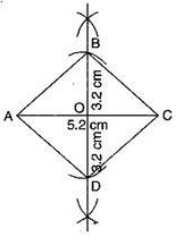(a) Draw AC = 5.2 cm and draw perpendicular bisectors on AC.
(b) Since, diagonals bisect at mid-point O, therefore get half of 6.4 cm, i.e., 3.2 cm.
(c) Draw two arcs on both sides of AC of radius 3.2 cm from intersection point O, which intersects at B and D.
(d) Join AB, BC, CD and DA.
It is required rhombus ABCD.

Question 3.

A rectangle with adjacent sides of length 5 cm and 4 cm.

Solution :

Given:; MN = 5 cm and MP = 4 cm.
To construct: A rectangle MNOP
Steps of construction: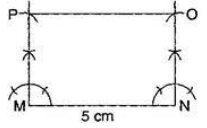(a) Draw a segment MN = 5 cm.
(b) At points M and N, draw perpendiculars of lengths 4 cm and produce them.
(c) Taking centres M and N, draw two arcs of 4 cm each, which intersect P and Q respectively.
(d) Join side PO.
It is required rectangle MNOP.

Question 4. A parallelogram OKAY where
OK = 5.5 cm and KA = 4.2 cm.

Solution :

Given:; OK = 5.5 cm and KA = 4.2 cm.
To construct: A parallelogram OKAY.
Steps of construction: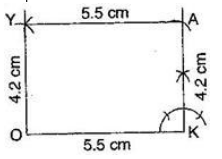(a) Draw a line segment OK = 5.5 cm.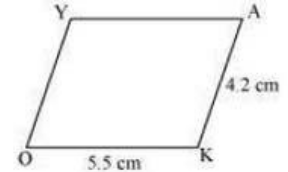(b) Draw an angle of; at K and draw an arc of radius KA = 4.2 cm, which intersects at point A.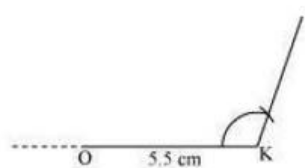(c) Draw another arc of radius AY = 5.5 cm and at point O, draw another arc of radius 4.2 cm which intersect at Y.

(d) Join AY and OY.
It is the required parallelogram OKAY.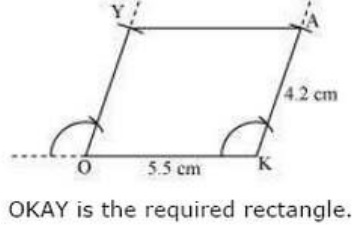### NCERT Solutions for Class 8 Maths Exercise 4.5

Draw the following:
Question 1.

The square READ with RE = 5.1 cm.

Solution :

Given:; RE = 5.1 cm.
Steps of construction: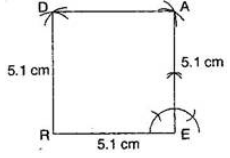(i) Draw RE = 5.1 cm.
(ii) At point E, construct an angle of; 90and draw an arc of radius 5.1 cm, which intersects at point A.
(iii) At point R, draw an arc of radius 5.1 cm at point A, draw another arc of radius 5.1 cm which intersects the first arc at point D.
It is the required square READ.

Question 2.

A rhombus whose diagonals are 5.2 cm and 6.4 cm.

Solution :

Given:; Diagonals of a rhombus
AC = 5.2 cm and BD = 6.4 cm.
To construct: A rhombus ABCD.
Steps of construction: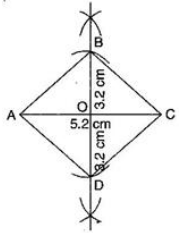(a) Draw AC = 5.2 cm and draw perpendicular bisectors on AC.
(b) Since, diagonals bisect at mid-point O, therefore get half of 6.4 cm, i.e., 3.2 cm.
(c) Draw two arcs on both sides of AC of radius 3.2 cm from intersection point O, which intersects at B and D.
(d) Join AB, BC, CD and DA.
It is required rhombus ABCD.

Question 3.

A rectangle with adjacent sides of length 5 cm and 4 cm.

Solution :

Given:; MN = 5 cm and MP = 4 cm.
To construct: A rectangle MNOP
Steps of construction: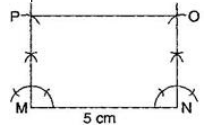(a) Draw a segment MN = 5 cm.
(b) At points M and N, draw perpendiculars of lengths 4 cm and produce them.
(c) Taking centres M and N, draw two arcs of 4 cm each, which intersect P and Q respectively.
(d) Join side PO.
It is required rectangle MNOP.

Question 4.

A parallelogram OKAY where
OK = 5.5 cm and KA = 4.2 cm.

Solution :

Given:; OK = 5.5 cm and KA = 4.2 cm.
To construct: A parallelogram OKAY.
Steps of construction: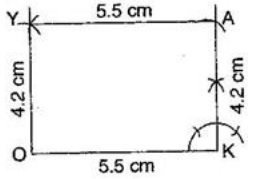(a) Draw a line segment OK = 5.5 cm.
(b) Draw an angle of 900 at K and draw an arc of radius KA = 4.2 cm, which intersects at point A.
(c) Draw another arc of radius AY = 5.5 cm and at point O, draw another arc of radius 4.2 cm which intersect at Y.
(d) Join AY and OY.
It is the required parallelogram OKAY

Get 30% off your first purchase!

X
error: Content is protected !!
Scroll to Top